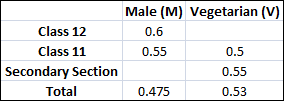### CAT 2007 Question Paper Question 40

Instructions

DIRECTIONS for the following four questions:

The proportion of male students and the proportion of vegetarian students in a school are given below. The school has a total of 800 students, 80% of whom are in the Secondary Section and rest equally divided between Class 11 and 12.Question 40

# What is the percentage of vegetarian students in Class 12?

Solution

There are 640 secondary section students, 80 11th class students and 80 12th class students.

If the fraction of vegetarians in 12th class is x, then

80x + 80*0.5 + 640*0.55 = 0.53*800

=> x + 0.5 + 4.4 = 5.3 => x = 0.4

=> 40% are vegetarians in class 12.